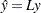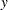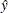Smoothing Matrix

When no iterative reweighting is done, the "Smoothing Matrix" denoted bydefines the linear relationship between the fitted and observed dependent variable values of a loess model. You can obtain the predicted values of a loess fit from the observed values viawhereis the vector of observed values andis the corresponding vector of predicted values of the dependent variable. Note thatis anbymatrix, whereis the number of observations in the analysis. PROC LOESS does not explicitly formif the DFMETHOD=EXACT option is not explicitly or implicitly selected.